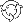The Theory \ Details \ table of symbols

# Table of symbolsVector of onesConfidence levelVector of instantaneous alphas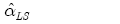Vector of ordinary least-squares estimates for instantaneous alphas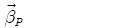Vector of portfolio betas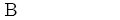Matrix of betas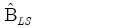Ordinary least-squares estimate for matrix of betasCertainty equivalent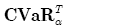Conditional Value-at-Risk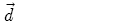Vector of dividend yieldsPortfolio dividend yield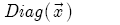Diagonal matrix with elements of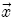at the main diagonal.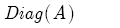Vector, whose elements are equal to diagonal elements of A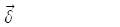Vector of transaction costs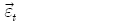Vector of regression residuals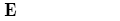Mathematical expectation symbolDownside volatilityNormalized downside volatility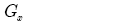Inaction region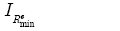Information ratioAbsolute risk aversion coefficientRelative risk aversion coefficientExpected simple rate of return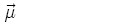Mu vectorExcess Mu vector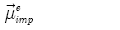Implied excess Mu vector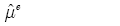Vector of sample estimates for excess Mu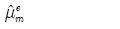Model-implied estimate for excess Mu vectorPortfolio Mu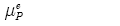Portfolio excess Mu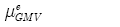Excess Mu for GMV portfolio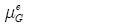Excess Mu for tangency portfolioSet of possible values for excess Mu vector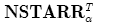Normalized STARR ratio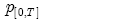Simple return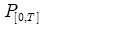Simple annual rate of return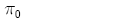Weight in riskless asset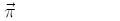Vector of portfolio weights, corresponding to risky assetsVector of portfolio weights for GMV portfolioVector of portfolio weights for tangency portfolio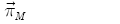Vector of portfolio weights for Merton portfolio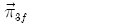Vector of portfolio weights for "three-fund" portfolio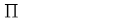Set of admissible portfolios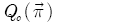Risk-adjusted expected excess rate of returnLogarithmic return, calculated on [0,T] period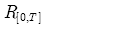Simple annual rate of return, calculated on [0,T] period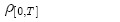Expected logarithmic rate of return, calculated on [0,T] period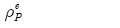Portfolio expected excess growth rate in the analytical model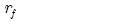Risk-free rateBorrowing rate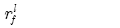Lending rate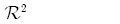Determination coefficient in regression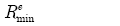Target excess growth rate, minimum acceptance excess rate (MAR)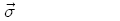Volatility vector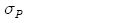Portfolio volatility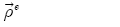Vector of expected excess growth rates in the analytical modelVolatility of GMV portfolio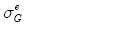Volatility of tangency portfolio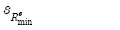Sortino ratioNormalized Sortino ratio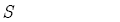Sharpe ratioInstantaneous Sharpe ratioSTARR ratio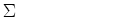Volatility matrixt-statistics vector for instantaneous alphas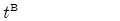t-statistics matrix for betas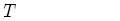Investment horizon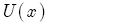Utility functionVector of assets relative contributions to portfolio variance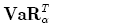Value-at-Risk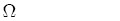Covariance matrix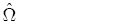Sample covariance matrix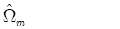Model-implied estimate of covariance matrixCovariance matrix for regression residuals